## MTH 241 - Homework #4 - Spring 2013

### SAMPLE SOLUTION: extra credit is available if you are the first to point out an error by midnight on Thursday, February 21st

require(mosaic)
options(digits = 3)
trellis.par.set(theme = col.mosaic())  # get a better color scheme for lattice
palette = trellis.par.get("superpose.symbol")$col # grab the palette  ### PROBLEMS TO TURN IN: #### Exercise 1 Low birth weight is an outcome that has been of concern to physicians for years. This is due to the fact that infant mortality rates and birth defect rates are very high for babies with low birth weight. A woman's behavior during pregnancy (including diet, smoking habits, and obtaining prenatal care) can greatly alter her chances of carrying the baby to term and, consequently, of delivering a baby of normal birth weight. The goal of the study was to identify risk factors associated with giving birth to a low birth weight baby. LBW = fetchData("LBW")  ## Data LBW found in package.  Hint: try “help(LBW)” for more information about the data set (including the units of measurement) a. Investigate the relationship between birth weight and the age of the mother. Make a scatterplot and show the least squares regression line on the plot. SAMPLE SOLUTION: xyplot(birthweight ~ age, data = LBW, xlab = "Age of mother (in yrs)", ylab = "Birth weight (in grams)", type = c("p", "r", "smooth"))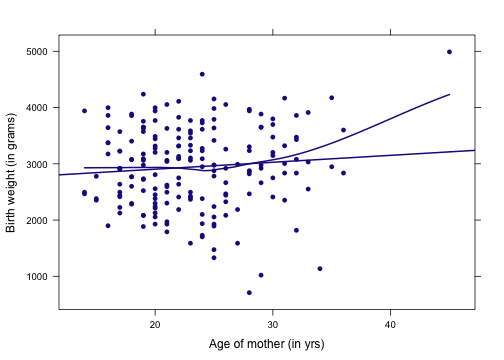There is a relatively weak linear relationship between the birth weight and the age of mother. This can be seen in the scatterplot by the relatively flat slope of the regression line. Also, the correlation between $$birthweight$$ and $$age$$ is 0.09, indicating a relatively weak association between the two variables. Finally, there is some concern about the rightmost point in the plot, which represents a large baby born to an older mother. This may be a point of high leverage, given its distance from the mean age of the mothers and its departure from the general pattern of the data. b. Fit a linear regression model for this relationship, and interpret the slope coefficient. SAMPLE SOLUTION: fm = lm(birthweight ~ age, data = LBW) coef(fm)  ## (Intercept) age ## 2657.3 12.4  The slope coefficient of 12.4 grams per year indicates that each additional year of a mother's age is associated with an expected increase in the birth weight of her child of 12.4 grams. #### Exercise 2 This problem continues the investigation of risk factors for low birth weight. a. Build a parallel slopes model for the same relationship, using the mother's smoking status as a conditioning variable. Interpret the coefficients from this model SAMPLE SOLUTION: fm2 = lm(birthweight ~ age + smoke, data = LBW) coef(fm2)  ## (Intercept) age smoke ## 2791.8 11.2 -276.3  The equation for our model is: $\hat{Birthweight} = 2791.8 + 11.2 \cdot age - 276.3 \cdot smoke$ The coefficients suggest that after controlling for a mother's smoking status, a one year increase in a mother's age will increase the predicted birth weight of her child by $$11.2$$ grams. However, given two mothers of the same age (any age), the birth weight of an infant whose mother smokes is expected to be $$276.3$$ grams less than the birth weight of an infant whose mother does not smoke. b. Construct a new scatterplot with smoking and non-smoking mothers distinguished by color. Add the parallel slopes model to your scatterplot. Hint: Use “makeFun()” and “plotFun()” SAMPLE SOLUTION: xyplot(birthweight ~ age, groups = smoke, xlab = "Age of mother (in yrs)", ylab = "Birth weight (in grams)", auto.key = TRUE, data = LBW) fit.bw = makeFun(fm2) palette = trellis.par.get("superpose.symbol")$col
plotFun(fit.bw(age, smoke = 0) ~ age, add = TRUE, col = palette)
plotFun(fit.bw(age, smoke = 1) ~ age, add = TRUE, col = palette)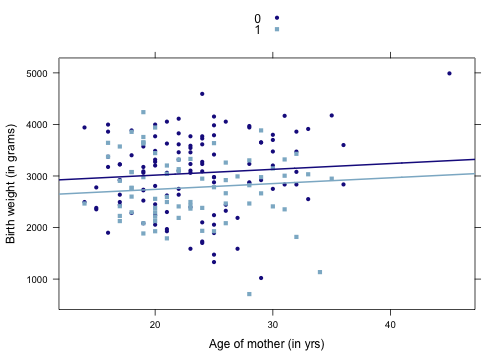c. Discuss the differences between the parallel slopes model and the simple linear regression model that you originally built.

SAMPLE SOLUTION: Although the SLR model has a slightly steeper slope ($$12.4$$) than the parallel slopes model ($$11.2$$), it does not distinguish between smoking mothers and non-smoking mothers. After conditioning on the mother's smoking status, we see that smoking is associated with a considerable decrease in the expected birth weight of baby. So while the overall relationship between the age of a mother and the birth weight of her baby remains similar in the two models, the parallel slopes model allows us to refine our estimates for two distinct groups of mothers (smokers and non-smokers).

#### Exercise 3

This problem continues the investigation of risk factors for low birth weight.

a. Now fit two separate models to the data: one model for smoking mothers and another model for non-smoking mothers. Interpret the coefficients for these models.

SAMPLE SOLUTION:

mod1 = lm(birthweight ~ age, data = subset(LBW, smoke == 0))
coef(mod1)

## (Intercept)         age
##      2408.4        27.6

mod2 = lm(birthweight ~ age, data = subset(LBW, smoke == 1))
coef(mod2)

## (Intercept)         age
##      3203.8       -18.8


Note that the same coefficients can be obtained using a single model with an interaction term (and in fact, this is the preferred formulation, for reasons that will become clear later):

mod3 = lm(birthweight ~ age * smoke, data = LBW)
coef(mod3)

## (Intercept)         age       smoke   age:smoke
##      2408.4        27.6       795.4       -46.4

3203.8 - 2795.4  # intercept for smokers

##  408

27.6 - 46.4  # slope for smokers

##  -18.8


The intercept for non-smokers of 2408 grams is the predicted birthweight for a newborn mom (this is obviously implausible). The slope of 27.6 grams for non-smokers indicates that we would expect an additional 27.6 grams of birthweight for one extra year of maternal age. The intercept and slope estimates for the smokers are 3204 and -18.8 grams, respectively.

Here, we observe a very different story than what the parallel slopes model was telling us. First, the sign of the coefficient for the age of the mother changes from positive to negative if the mother smokes. This suggests that among non-smoking mothers, greater age is associated with higher birth weight of children, whereas among mothers who smoke, the effect is reversed – greater age is associated with lower birth weight. Unlike in the parallel slopes model, where we forced the two groups to have the same relationship between birth weight and a mother's age, the greater flexibility offered by the interaction model allows the slope to change dramatically.

b. Plot the separate models on the scatterplot. Discuss the benefits and limitations of the parallel slopes model you built previously, as compared to the separate models you just built.

SAMPLE SOLUTION:

xyplot(birthweight ~ age, groups = smoke, xlab = "Age of mother (in yrs)", ylab = "Birth weight (in grams)",
data = LBW, auto.key = TRUE, type = c("p", "r"))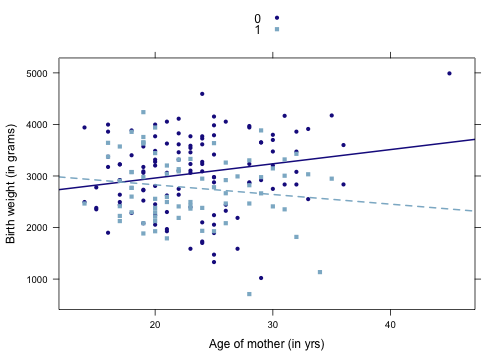The parallel slopes model has the advantage of being simpler, and forcing the relationship between birth weight and the age of a mother to be the same, both among smoking mothers and non-smoking mothers. This will be an advantage only if we truly believe that that relationship remains constant across the groups. If on the other hand, we believe that the two groups behave importantly different in this respect, then the interaction model will more appropriately reveal those differences.

c. Of the three attempts you have made to model the relationship between birth weight and a mother's age (i.e. 1) a single linear regression model; 2) a parallel slopes model conditioned on smoking status, and; 3) separate single linear regression models for smoking and non-smoking mothers), which do you think best captures the association present in the data? Justify your answer.

SAMPLE SOLUTION: The third model (the interaction model) seems to be the best. In particular, the parallel slopes model can do everything the SLR model can do and more, and similarly the interaction model can do everything the parallel slopes model can do, and more. Thus, if the best fit to the data was a parallel slopes model, than the coefficient of the interaction term would be close to zero. But that is not the case here.

#### Exercise 4

This problem continues the investigation of risk factors for low birth weight.

a. Build a simple linear model for birth weight as a function of the mother's weight, and interpret the coefficients.

SAMPLE SOLUTION:

xyplot(birthweight ~ momweight, xlab = "Weight of mother (in pounds)", ylab = "Birth weight (in grams)",
data = LBW, type = c("p", "r"))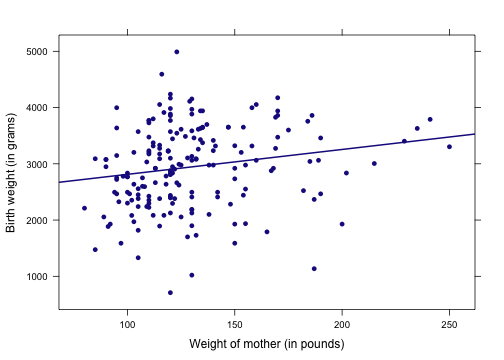fm3 = lm(birthweight ~ momweight, data = LBW)
coef(fm3)

## (Intercept)   momweight
##     2369.67        4.43


The coefficients suggest that each pound of a mother's weight is associated with an additional 4 grams in the expected birth weight of her child.

b. Add the conditioning variable $$hypertension$$ to your model. How does this affect the coefficients?

SAMPLE SOLUTION:

parallel = lm(birthweight ~ momweight + hypertension, data = LBW)
coef(parallel)

##  (Intercept)    momweight hypertension
##      2260.57         5.56      -600.02

interaction = lm(birthweight ~ momweight * hypertension, data = LBW)
coef(interaction)

##            (Intercept)              momweight           hypertension
##                2342.68                   4.92               -1270.46
## momweight:hypertension
##                   4.38


xyplot(birthweight ~ momweight, groups = hypertension, xlab = "Age of mother (in yrs)",
ylab = "Birth weight (in grams)", data = LBW, type = c("p", "r"))

fit.parallel = makeFun(parallel)
plotFun(fit.parallel(momweight, hypertension = 0) ~ momweight, add = TRUE, col = palette)
plotFun(fit.parallel(momweight, hypertension = 1) ~ momweight, add = TRUE, col = palette)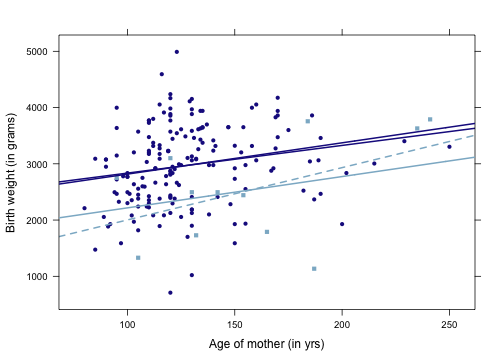In this case the large negative coefficient for $$hypertension$$ suggests that hypertension is associated with a 600 gram decrease in the birth weight of a child born to a mother with hypertension. Note that both models are similar in this case, so we may prefer the simpler parallel slopes model.

c. Examine the residuals from the parallel slopes model and determine whether the assumptions that the residuals are random and normally distributed are reasonably met. At a minimum, you'll want to consider:

• the distribution of the residuals
• the relationship between the residuals and the predicted values
• the relationship between the residuals and the explanatory variable

SAMPLE SOLUTION:

xhistogram(~resid(parallel), fit = "normal")

## Loading required package: MASS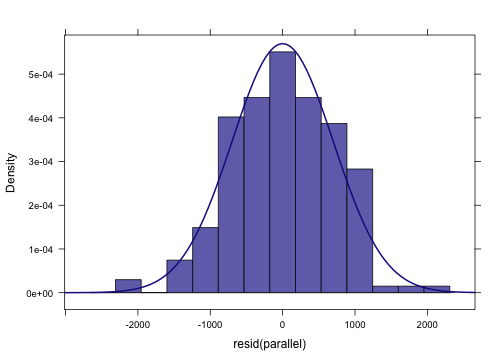plot(parallel, which = 1)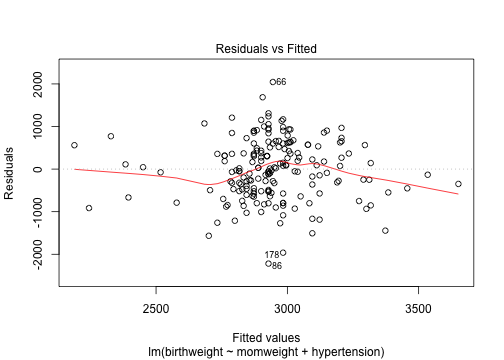plotPoints(resid(parallel) ~ momweight, xlab = "mom weight (pounds)", type = c("p",
"r", "smooth"), data = LBW)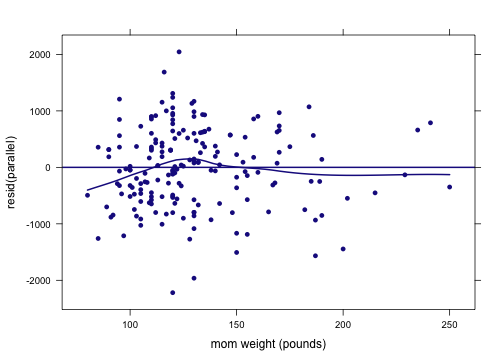These plots look fairly reasonable. In the density plot, we can see the the residuals appear to be approximately normally distributed. In the second plot, we see that there is no serious deviation (nonlinearity), with the exception of the range from 2800 to 3000 grams from the predicted value plot and mom weights less than 150 pounds. This suggests that the assumption of linearity is not unreasonable. Moreover, there is little evidence of heteroskedasticity, since the vertical spread of the residuals seems to be relatively constant across different values of the mother's weight, as well as the fitted birth weights.